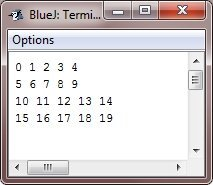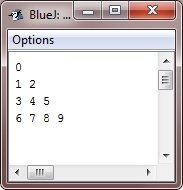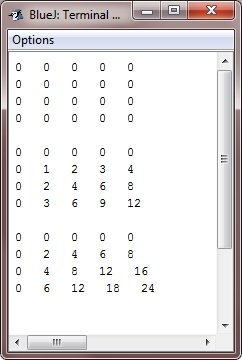# Java Multidimensional Array

A multidimensional array in Java is an array of arrays, with each element being another array.

A Java two-dimensional array is a collection of one-dimensional arrays; a three-dimensional array is a collection of two-dimensional arrays; and so on.

## Java Two-Dimensional Array

To declare a multidimensional array variable, determine each additional index using another set of square brackets. For instance, the below code fragment declares a two-dimensional array variable called twoD.

`int[][] twoD = new int;`

This creates a four-by-five array and then assigns it to two dimensions. Actually, this matrix is made as an array of arrays of int.

The following program numbers each element present in the array from left to right and top to bottom, and then shows these values.

```public class JavaProgram
{
public static void main(String args[])
{
int twoD[][] = new int;
int i, j, k = 0;

for(i=0; i<4; i++)
{
for(j=0; j<5; j++)
{
twoD[i][j] = k;
k++;
}
}

for(i=0; i<4; i++)
{
for(j=0; j<5; j++)
{
System.out.print(twoD[i][j] + " ");
}
System.out.println();
}
}
}```

When the above Java program is compiled and executed, it will produce the following output:When you allocate the memory for a multidimensional array, then you only need to specify the memory for the first (leftmost) dimension. You can also allocate the remaining dimensions separately. For instance, the code given below allocates the memory for the first dimension of twoD when it is declared. It allocates the second dimension manually.

```int[][] twoD = new int[];
twoD = new int;
twoD = new int;
twoD = new int;
twoD = new int;```

Although there is no advantage to allocating the second-dimension arrays individually in this case, there may be in others. When allocating dimensions manually, for example, you do not have to assign the same number of elements to each dimension. As previously stated, because multidimensional arrays are arrays of arrays, you have control over the length of each array. For example, the following program generates a two-dimensional array with unequal second dimension sizes:

```public class JavaProgram
{
public static void main(String args[])
{
int[][] twoD = new int[];
twoD = new int;
twoD = new int;
twoD = new int;
twoD = new int;

int i, j, k = 0;

for(i=0; i<4; i++)
{
for(j=0; j<i+1; j++)
{
twoD[i][j] = k;
k++;
}
}

for(i=0; i<4; i++)
{
for(j=0; j<i+1; j++)
{
System.out.print(twoD[i][j] + " ");
}
System.out.println();
}
}
}```

When the above Java program is compiled and executed, it will produce the following output:In Java, it is possible to initialize multidimensional arrays. To do so, simply enclose each dimension's initializer in its own set of curly braces.

The following program creates a matrix where each element holds the product of row and column indexes. Notice here that you can also use expressions as well as literal values inside of array initializers.

```public class JavaProgram
{
public static void main(String args[])
{
double[][] m = {
{ 0*0, 1*0, 2*0, 3*0 },
{ 0*1, 1*1, 2*1, 3*1 },
{ 0*2, 1*2, 2*2, 3*2 },
{ 0*3, 1*3, 2*3, 3*3 }
};
int i, j;

for(i=0; i<4; i++)
{
for(j=0; j<4; j++)
{
System.out.print(m[i][j] + "");
}
System.out.println();
}
}
}```

When the above Java program is compiled and executed, it will produce the following output:As you can see here, each row in the array is initialized as specified in the initialization lists.

## Java Three-Dimensional Array

Now, let's look at this example that uses a multidimensional (three-dimensional) array. The following program creates a 3-by-4-by-5 three-dimensional array. This then loads each element with the product of its indexes. Finally, it displays these products.

Here is an example program that demonstrates the concept and use of three-dimensional arrays in Java:

```public class JavaProgram
{
public static void main(String args[])
{
int threeD[][][] = new int;
int i, j, k;

// initializing a three-dimensional array
for(i=0; i<3; i++)
{
for(j=0; j<4; j++)
{
for(k=0; k<5; k++)
{
threeD[i][j][k] = i * j * k;
}
}
}

// displaying three-dimensional arrays
for(i=0; i<3; i++)
{
for(j=0; j<4; j++)
{
for(k=0; k<5; k++)
{
System.out.print(threeD[i][j][k] + "");
}
System.out.println();
}
System.out.println();
}
}
}```

When the above Java program is compiled and executed, it will produce the following output:Java Online Test

« Previous Tutorial Next Tutorial »